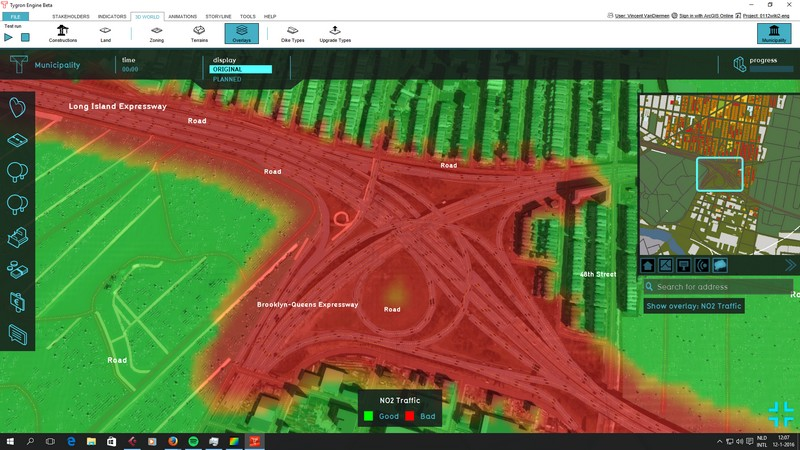# Traffic NO2(Overlay)

(diff) ← Older revision | Latest revision (diff) | Newer revision → (diff)

The Traffic NO2 Overlay is an overlay which displays the impact of traffic air polution (in μg/m³ NO2) in different colors for the project area. These colors indicate traffic air polution of a location based on traffic flow and distance.
It becomes available for all stakeholders when added to a project.Optional Traffic NO2 (Air polution effect) overlay

## Calculations

The calculations follow the standard rekenmethode 1 (SRM 1), as described on :

Cjm[NO2] = Ca,jm[NO2] + Cb,jm[NO2]

where:

• Cjm[NO2] = annual average concentration [μg/m3]
• Ca,jm[NO2] = annual average background-concentration (default = 0 [μg/m3], can be adjusted via the overlay attributes)
• Cb,jm[NO2] = annual average traffic contribution to NO2 concentration [μg/m3]

To compute the annual average traffic contribution to NO2, Cb,jm[NO2] [μg/m3], the following formula from SRM1 is applied:

Cb,jm[NO2]= fNO2 * Cb,jm[NOx] + (B*Ca,jm[O3]*Cb,jm[NOx]*(1-fNO2))/(Cb,jm[NOx]*(1-Fno2)+K)

where:

• Cb,jm[NOX] = annual average traffic contribution to NOx concentration [μg/m3]
• Cb,jm[O3] = annual average concentration ozon [μg/m3] (default = 42)
• fNO2 = weighted fraction direct emitted NO2 [-]
• B = parameter (default 0.6)
• K = parameter for conversion NO to NO2 (default = 100 μg/m3)

To compute the annual average traffic contribution to NOX, Cb,jm[NOX] [μg/m3], the following formula from SRM1 is applied:

Cb,jm[NOx] = Fk * ENOx * θ * Fb * Fmeteo

where:

• Fk = calibration factor [-] (default = 0.62]
• ENOx = emission number NOx [μg/m/s]
• θ = delution factor [-]
• Fb = tree factor (default = XX)
• Fmeteo = 5/windspeed. Default value for windspeed is 5 m/s

The delution factor, θ, is computed till a distance of 30m with:

θ = a * S^2 + b * S + c

And from 30-60m (for road-type 1 and 4 only):

θ = alpha * S^-0.747

where:

• S is the calculation distance
• a, b, c and alpha are parameters depend on road-type:

The weighted fraction direct emitted NO2 [-] is computed as:

fNO2 = ENO2/ENOx

where:

• ENO2 = emission number NOs [μg/m/s]

The emission numbers (E) for both NOx and NO2 are computed as:

E = N * [(1-FS)*((1-(fm+fz+fb))*El + fm * Em + fz * Ez + fb * Eb) + FS * ((1-(fm + fz + fb)) * El,d + Fm * Em,d + fz * Ez,d+fb*Eb,d)]*1000/(24*3600)

where:

• N = traffic intencity [units per day]
• fm = fraction vans [-]
• fz = fraction trucks [-]
• fb = fraction busses [-]
• El = emission value cars [g/km]
• Em = emission value vans [g/km]
• Ez = emission value trucks [g/km]
• Eb = emission value busses [g/km]
• FS = fraction congested traffic [-]
• E*,d = emission factor per class (cars, vans, trucks or busses) if congested